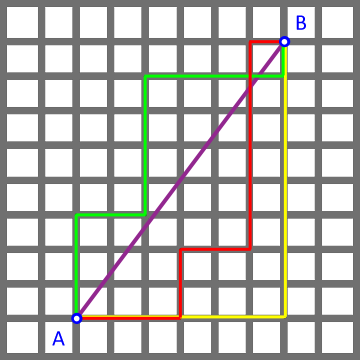## The Taxicab Metric

In a certain sense, the distance between two points could be thought of length of the shortest path between those two points. This, at least, agrees with our normal intuition about the distance between points stemming from the space in which we live. There are some natural consequences of this interpretation, of course -- for instance:

• The length of the shortest path between two points should be zero if the two points are really the same point, and greater than zero if they are distinct points.

• This length of the shortest path from a point $a$ to another point $b$ should be the same as the length of the shortest path from $b$ to $a$.

• The length of any path from $a$ to $b$ that passes through some point $c$ could be equal to the length of the shortest path from $a$ to $b$, or it might be longer (but it will never be shorter).

If we let $d(a,b)$ represent the distance between points $a$ and $b$, then we can write the properties above more succinctly as:

• $d(a,b) \ge 0$ (with equality if and only if $a=b$)
• $d(a,b) = d(b,a)$
• $d(a,c) + d(c,b) \ge d(a,b)$

To attach some formal verbiage to this, we call any such function $d$ that has the three properties above a metric.

Of course, if $p$ and $q$ are points in a coordinate plane with coordinates $(x_p,y_p)$ and $(x_q, y_q)$, respectively, and we appeal to the usual definition for the distance between two points, inspired by the Pythagorean Theorem

$$d(p,q) = \sqrt{(x_q-x_p)^2 + (y_q-y_p)^2}$$

we are not surprised to find that all three of the aforementioned properties are satisfied.

However, is the distance formula above the only one that satisfies these properties?

Suppose you live in a city where all of the roads are arranged in a grid-like pattern, running north and south, or east and west. Perhaps you wish to have a taxi drive you from point A to point B which is 6 blocks east and 8 blocks north of point A. As it relates to computing your overall fare, what will the taxi driver claim as the distance from point A to point B?Unless your taxi can sprout wings and take you directly from A to B (following the path shown in purple above), the taxi driver will probably charge you for more than $\sqrt{6^2 + 8^2} = 10$ blocks! Indeed, while there are several minimal paths the taxi driver could take to get you from A to B (e.g., the red, green, and yellow paths shown), they all appear to have length $6+8 = 14$ blocks.

Considering other possible starting points and destinations quickly leads one to conclude that the "distance formula" the cab driver uses to calculate the distance (in blocks) between $p$ and $q$ is given by

$$d_T(p,q) = |x_q - x_p| + |y_q - y_p|$$

Now the question is: "Is $d_T$ a metric?" Does $d_T$ satisfy those three properties previously discussed?

The first two properties are trivially true, so it remains to demonstrate the third.

Suppose we have three points $a$, $b$, and $c$, with coordinates $(x_a,y_a)$, $(x_b,y_b)$, and $(x_c,y_c)$, respectively. We need to show that $d_T(a,c) + d_T(c,b) \ge d_t(a,b)$.

Recalling that for any real values $s$ and $t$ we have $|s| + |t| \ge |s+t|$, consider the following:

$$\begin{array}{rcl} d_T(a,c) + d_T(c,b) &=& |x_c-x_a| + |y_c-y_a| + |x_b - x_c| + |y_b - y_c|\\ &=& |x_c - x_a| + |x_b - x_c| + |y_c-y_a| + |y_b-y_c|\\ &\ge& |x_c-x_a+x_b-x_c| + |y_c-y_a+y_b-y_c|\\ &=& |x_b-x_a| + |y_b - y_a|\\ &=& d_T(a,b) \end{array}$$

which is what we needed to show. As such, $d_T$ is indeed a metric.